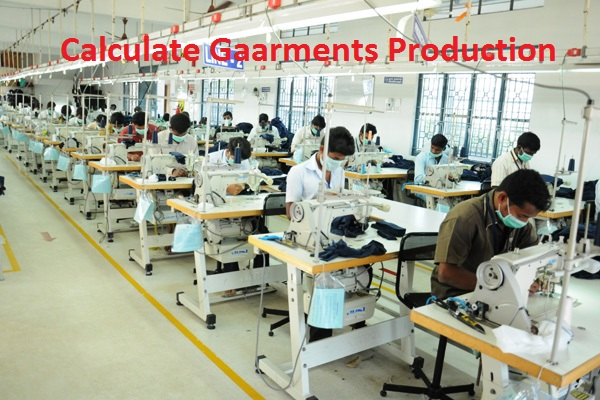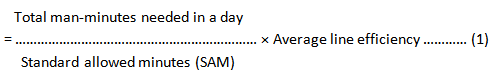# How to Calculate Garments Production?

### How to Calculate Garments Production?

Calculation of garment production plays an important role in shipping the goods timely. Estimation calculation is not the tough task that we normally seemed. As its importance on garments business, today I will present the estimation system of garments production in this article.Calculation of Garments Production

#### Calculation of Garments Production:

There are some points which must be needed during the calculation of garments production, Those are-

1. The standard allowed minutes (SAM),
2. Number of operators- working in a line,
3. Number of hours running-production line work in a day,
4. Average line efficiency,
5. Total break time including Launch, Tea, and Others.

During the calculation of garments production, we have to follow a formula, that is-

Per day production (pc),Here, total man-minutes mean the number of operators working in a day including total break time for Launch, Tea, and Others.

Or,

Total man-minutes = [Total Number of operator’s × {(Working hour in a day × 60minutes) – Total break time}]

Now, we can write equation (1) in the following way-

Per day production (Pc),So, it is the main formula for estimation/calculation of garment production.

Now, one example is enough to understand the estimation of garment production and that is in the below:

#### Example:

Let,
For a polo shirt garment,

• The standard allowed minutes (SAM) is-25 minutes,
• Number of operators in a working line is-20
• Number of hours running-production line work in a day- 8hrs
• Average line efficiency – 60%
• Total break time including Launch, Tea, and Others- 60 minutes

So, calculate the estimation of polo shirt garment production.

Solution:
Now, applying the above information in equation (2), we have-

Per day production (pc),= 336 × 60%
= 201pcs

So, the Estimation of polo shirt garment production per day is 201pcs.

Note:

Calculate the below problem by following the above formula:

Calculate the clothing production of a polo shirt order from H&M by following some key information like Standard allowed minutes- 23 minutes, total no. of operators working in a sewing line 8hrs in a day- 15, line efficiency- 55% and total break time are 1hrs.

•kunal kaspale

Thnxx

•Mayedul

U r always wc dear……………

•safy

Usually planning will be done for working hrs only, not including break time, so we can take working minutes directly, no need to deduct break time. For example let us consider factory timing is 9 am to 6pm, and break time is 1 pm to 2 pm.
So we can calculate directly, ie. No. Of operators (20) x Working mins (8×60) x line eff (60%) / 25 SAM = 230 pcs

•Mayedul

Thanks for sharing your valuable experience……….
keep in touch…………

•Sohel

Pls explain SAM.

•Mayedul

SAM= Standard allowed minute

•Sohel

Pls explain SAM & line efficiency.

•Mayedul

Keep in touch………..Those articles are coming………….

•Puttappa

Important articles for further seekers.

•Mayedul Islam

•Ritesh Agarwal

hi. please send me more details im mail with above full methodology and Q &A
Rgds

•Amjad

Hi,

•shohag

how to calculate factory capacity & production?

•shohag

what is takt time ? why is so important for IE ?

•Robin

•Robin
•Suresh